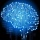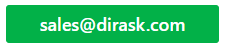Home
IT Knowledge
IT Career
Inspiration
Languages
EN

# JavaScript - random int in range

4 points
Created by:Root-ssh
69140

Hi, today I would like to show you how to generate random integer number in given range in JavaScript.

Below we have quick solution how we can solve this problem. Each time click 'Run' button we will see different results (statistically).

## Random int between 0 and 2

``````// ONLINE-RUNNER:browser;

/*
inclusive min (result can be equal to min value)
inclusive max (result can be equal to max value)
*/
function randomizeInteger(min, max) {
return min + Math.floor((max - min + 1) * Math.random());
}

// Example:

console.log(randomizeInteger(0, 2)); // 0
console.log(randomizeInteger(0, 2)); // 2
console.log(randomizeInteger(0, 2)); // 1
console.log(randomizeInteger(0, 2)); // 1``````

If we want to change the range, we just need to call this method with our descired min and max.

About min and max value as stated in commnet:

• inclusive min (result can be equal to min value)
• inclusive max (result can be equal to min value)